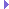GeomVertexAnimationSpec Class Reference

This object describes how the vertex animation, if any, represented in a GeomVertexData is encoded. More...

Inheritance diagram for GeomVertexAnimationSpec:## Public Member Functions

__init__ ()

__init__ (const GeomVertexAnimationSpec other)

GeomEnums::AnimationType getAnimationType ()
Returns the type of animation represented by this spec. More...

bool getIndexedTransforms ()
This is only meaningful for animation_type AT_hardware. More...

int getNumTransforms ()
This is only meaningful for animation_type AT_hardware. More...

GeomVertexAnimationSpec operator= (const GeomVertexAnimationSpec other)

output (Ostream out)

setHardware (int num_transforms, bool indexed_transforms)
Specifies that vertex animation is to be performed by the graphics hardware (or at least by the graphics backend API, which is actually still free to animate the vertices on the CPU). More...

setNone ()
Specifies that no vertex animation is represented by this spec. More...

setPanda ()
Specifies that vertex animation is to be performed by Panda. More...Public Member Functions inherited from GeomEnums
__init__ ()

__init__ (const GeomEnums)

## Public Attributes

GeomEnums::AnimationType animation_type
Returns the type of animation represented by this spec. More...

bool indexed_transforms
This is only meaningful for animation_type AT_hardware. More...

int num_transforms
This is only meaningful for animation_type AT_hardware. More...

## Additional Inherited MembersPublic Types inherited from GeomEnums
enum  AnimationType { AT_none = 0, AT_panda = 1, AT_hardware = 2 }

enum  Contents {
C_other = 0, C_point = 1, C_clip_point = 2, C_vector = 3,
C_texcoord = 4, C_color = 5, C_index = 6, C_morph_delta = 7,
C_matrix = 8, C_normal = 9
}

enum  GeomRendering {
GR_indexed_point = 1, GR_indexed_other = 65536, GR_indexed_bits = 65537, GR_point = 2,
GR_point_uniform_size = 4, GR_per_point_size = 8, GR_point_perspective = 16, GR_point_aspect_ratio = 32,
GR_point_scale = 64, GR_point_rotate = 128, GR_point_sprite = 256, GR_point_sprite_tex_matrix = 512,
GR_point_bits = 1022, GR_triangle_strip = 1024, GR_triangle_fan = 2048, GR_line_strip = 4096,
GR_composite_bits = 7168, GR_strip_cut_index = 131072, GR_flat_first_vertex = 8192, GR_flat_last_vertex = 16384,
GR_shade_model_bits = 24576, GR_render_mode_wireframe = 262144, GR_render_mode_point = 524288, GR_adjacency = 1048576
}

enum  NumericType {
NT_uint8 = 0, NT_uint16 = 1, NT_uint32 = 2, NT_packed_dcba = 3,
NT_packed_dabc = 4, NT_float32 = 5, NT_float64 = 6, NT_stdfloat = 7,
NT_int8 = 8, NT_int16 = 9, NT_int32 = 10, NT_packed_ufloat = 11
}

enum  PrimitiveType {
PT_none = 0, PT_polygons = 1, PT_lines = 2, PT_points = 3,
PT_patches = 4
}

enum  ShadeModel { SM_uniform = 0, SM_smooth = 1, SM_flat_first_vertex = 2, SM_flat_last_vertex = 3 }

enum  UsageHint {
UH_client = 0, UH_stream = 1, UH_dynamic = 2, UH_static = 3,
UH_unspecified = 4
}

## Detailed Description

This object describes how the vertex animation, if any, represented in a GeomVertexData is encoded.

Vertex animation includes soft-skinned skeleton animation and morphs (blend shapes), and might be performed on the CPU by Panda, or passed down to the graphics backed to be performed on the hardware (depending on the hardware's advertised capabilities).

Changing this setting doesn't by itself change the way the animation is actually performed; this just specifies how the vertices are set up to be animated.

## ◆ __init__() [1/2]

 __init__ ( )

## ◆ __init__() [2/2]

 __init__ ( const GeomVertexAnimationSpec other )

## ◆ getAnimationType()

 GeomEnums::AnimationType getAnimationType ( )

Returns the type of animation represented by this spec.

## ◆ getIndexedTransforms()

 bool getIndexedTransforms ( )

This is only meaningful for animation_type AT_hardware.

If true, it indicates that the format uses indexed animation tables. It is false if each vertex will reference the first _num_transforms table entries only.

## ◆ getNumTransforms()

 int getNumTransforms ( )

This is only meaningful for animation_type AT_hardware.

It specifies the maximum number of transforms that might be simultaneously applied to any one vertex by the data in this format.

## ◆ operator=()

 GeomVertexAnimationSpec operator= ( const GeomVertexAnimationSpec other )

## ◆ output()

 output ( Ostream out )

## ◆ setHardware()

 setHardware ( int num_transforms, bool indexed_transforms )

Specifies that vertex animation is to be performed by the graphics hardware (or at least by the graphics backend API, which is actually still free to animate the vertices on the CPU).

This is only legal if the graphics hardware can support the specified limits on number of transforms and/or indexed transforms. Also, no current graphics API's support morphing.

## ◆ setNone()

 setNone ( )

Specifies that no vertex animation is represented by this spec.

## ◆ setPanda()

 setPanda ( )

Specifies that vertex animation is to be performed by Panda.

This is the most general setting and can handle any kind of vertex animation represented.

## ◆ animation_type

 GeomEnums::AnimationType animation_type

Returns the type of animation represented by this spec.

## ◆ indexed_transforms

 bool indexed_transforms

This is only meaningful for animation_type AT_hardware.

If true, it indicates that the format uses indexed animation tables. It is false if each vertex will reference the first _num_transforms table entries only.

## ◆ num_transforms

 int num_transforms

This is only meaningful for animation_type AT_hardware.

It specifies the maximum number of transforms that might be simultaneously applied to any one vertex by the data in this format.